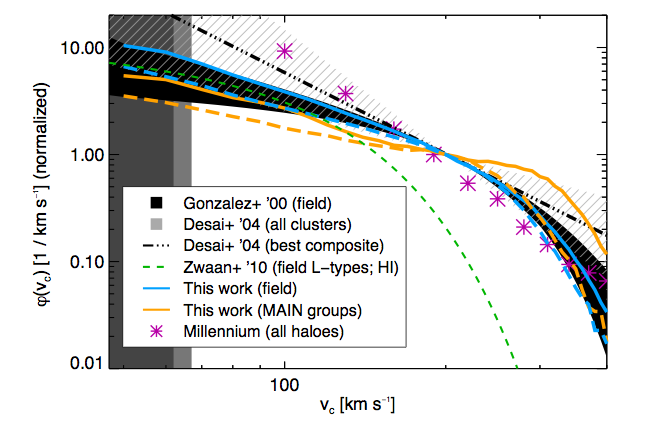# Circling problems with our model of the Universe: what can groups of galaxies tell us about Lambda CDM?

Is our picture of the Universe right?

Lambda CDM is successful but doesn’t predict some important things right—-stellar mass or luminosity functions, for instance.  But these involve a lot of baryon physics we might not know—so astrophysicists look at things that are bigger, and involve only gravity, which would be insensitive to feedback processes, etc.  Simulations suggest a circular velocity function (CVF) with power law -3 to -4—i.e., CVF ~v^-(3-4), v the velocity. The CVF is simply the number density of galaxies per unit circular velocity: how many galaxies are there that have a given speed of stars, etc, whirling about their center? However, for galaxies that are isolated, observations show shallower slopes.  However, in clusters of galaxies, the slope is roughly consistent with simulation (-2.4 +/- .8).  So where does the transition occur?  The authors look at groups using Sloan Digital Sky Survey (SDSS).

How do you tell?

How do you find velocities? There are two basic ways to go: spectroscopic and photometric.  In most areas of astrophysics spectroscopic (matching absorption and emission lines’ shifts in different objects’ spectra) is considered more accurate, but here the authors use photometric for two reasons I’ll describe.  Photometric is using the total light received, rather than matching specific spectral lines.

The authors use two separate photometric methods.  First, they use the so-called inverse Fundamental Plane relation and Tully-Fisher relation.  The Fundamental Plane is for elliptical galaxies and relates the half-light radius (radius out to which 1/2 the light of the galaxy is included) to the velocity dispersion and surface brightness. So, the Fundamental Plane relates three parameters: size, brightness, and velocity dispersion.  Thus, if the radius and surface brightness are known, velocity dispersion can be found.  The Tully-Fisher relation essentially does the same thing but for spiral galaxies, connecting the circular velocity to the luminosity.  So if luminosity is known, circular velocity can be found.

Second, the authors use a simple (ish) relationship between the total mass in stars in a galaxy and the circular velocity (basically, v=(2GM/r)^(1/2), with a bit more complexity added).  This just comes from balancing gravity with centrifugal force, though there are details that come in to get it more accurate which I won’t describe here.

Why did the authors avoid spectroscopic methods? First, the SDSS measurements they use have a cutoff on the velocity dispersion below which this method stops working.  Second, the spectroscopic measurements are from stars absorption and emission of light—but whether the velocity derived is the same as that a test particle in the gravitational potential of a rotationally-supported galaxy would actually have is an open question.Circular velocity functions (CVFs) from several different papers; the authors’ is in orange. Notice it is shallower than the purple starred line, which is from a big N-body simulation called Millenium. This shows how Lambda CDM predictions for the CVF disagree with observation. From the paper.

So . . . is Lambda CDM a winner?

The authors find agreement with other previous work on groups—that the slope of the CVF is shallower than simulations predict, and more similar to that for isolated galaxies’ CVFs than that for clusters’ CVFs.  In concluding, the authors mention that HI measurements of the CVF—in other words, using neutral hydrogen gas emission to derive it—provide a good cross check on their work.  Two other points are also worth noting: the CVF shape is independent of the mass of the dark matter halo up to M_halo ~10^15 M_Sun, and the CVF shape is mainly determined by the mixture of early and late type galaxies in each group.